# Entropy

An information-theoretical measure of the degree of indeterminacy of a random variable. Ifis a discrete random variable defined on a probability space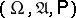and assuming values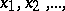with probability distribution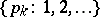,, then the entropy is defined by the formula(1)

(here it is assumed that). The base of the logarithm can be any positive number, but as a rule one takes logarithms to the base 2 or, which corresponds to the choice of a bit or a nat (natural unit) as the unit of measurement.

Ifandare two discrete random variables taking valuesand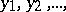with probability distributionsand, and ifis the conditional distribution ofassuming that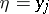,then the (mean) conditional entropyofgivenis defined as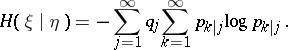(2)

Letbe a stationary process with discrete time and discrete space of values such that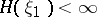. Then the entropy (more accurately, the mean entropy)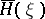of this stationary process is defined as the limit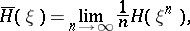(3)

where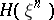is the entropy of the random variable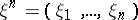. It is known that the limit on the right-hand side of (3) always exists and that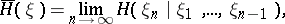(4)

where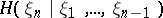is the conditional entropy of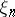given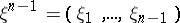. The entropy of stationary processes has important applications in the theory of dynamical systems.

Ifandare two measures on a measurable space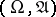and ifis absolutely continuous relative toand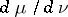is the corresponding Radon–Nikodým derivative, then the entropyofrelative tois defined as the integral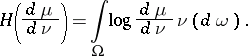(5)

A special case of the entropy of one measure with respect to another is the differential entropy.

Of the many possible generalizations of the concept of entropy in information theory one of the most important is the following. Letand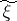be two random variables taking values in certain measurable spaces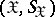and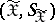. Suppose that the distribution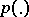ofis given and letbe a class of admissible joint distributions of the pair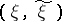in the set of all probability measures in the product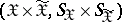. Then the-entropy (or the entropy for a given conditionof exactness of reproduction of information (cf. Information, exactness of reproducibility of)) is defined as the quantity(6)

where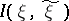is the amount of information (cf. Information, amount of) ingivenand the infimum is taken over all pairs of random variablessuch that the joint distribution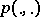of the pairbelongs toandhas the distribution. The classof joint distributionsis often given by means of a certain non-negative measurable real-valued function,,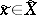, a measure of distortion, in the following manner: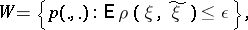(7)

whereis fixed. In this case the quantity defined by (6), whereis given by (7), is called the-entropy (or the rate as a function of the distortion) and is denoted by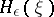. For example, if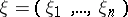is a Gaussian random vector with independent components, if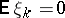,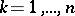, and if the function,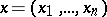,, has the formthencan be found by the formula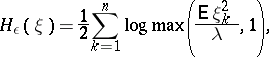whereis defined byIfis a discrete random variable, ifandare the same, and ifhas the formthen the-entropy for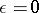is equal to the ordinary entropy defined in (1), that is,.